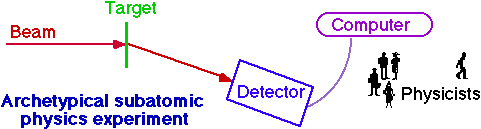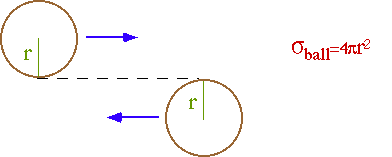Experimental Subatomic Physics

* Some subatomic physics experiments look in nature to study naturally occurring subatomic processes

* but most experiments usually bang things together to see:

• how big they are,
• what they are made of,
• how they interact,
• how they can be excited, and
• what new particles are produced.

* In order to extract this information from experiments,

• We need to know:
- what we are banging together,
- their energy, and
- their luminosity.
• We need to measure
- the types of particles produced in the collision,
- their energies,
- their angles and point of origin.

* After analysis, the results are expressed as

• production cross sections
• particle masses, excitation energies
* Theoretical ideas are tested by comparing predictions and observations.

The first two subatomic particles to be discovered were the electron and the atomic nucleus. The concepts used in their discoveries are still used today - just vastly scaled up in energy, size, money, and people.

Thompson's discovery of the electron was the first example of a non-accelerator particle search experiment. Thompson showed that cathode rays were electrically charged particles with a fixed value of e /m. Since e was roughly known, he showed that the cathode particles were ~2000 times lighter than a hydrogen atom. Atoms were considered to be the indivisible fundamental constituents of matter, so nothing lighter was expected to exist.

Rutherford's discovery of the atomic nucleus was the prototype for all subsequent high energy physics experiments.Rutherford CDF team Geiger(RA),   Marsden(UG) cast of thousands beam a particles   (Rd, ~5 MeV) antiprotons/protons   (Tevatron, 900 GeV) target foil (Au,Ag) proton/antiproton beam detector ZnS screen + eyeball 10000 ton hermetic   detector data acquisition pencil networks, workstations, disks, tapes, .... analysis student+pencil ~102 students + computers

Starting with ordinary matter (electrons, nucleons, photons), we want to probe smaller and smaller distance scales and produce new states of matter.

We use accelerators to produce our high energy probes, and detectors to observe what happens.

Accelerators

electromagnetic energy (electromagnetic fields, waves)

Þ energetic (charged) particles

Detectors

energetic (charged) particles

Þ electromagnetic energy (light, charge)

In order to do physics, an accelerator must provide us with:

(1) energy
l=hc/E ,  E>2m
(2) luminosity
Rate (s-1) = s (cm-2) L (cm-2 s-1)

The cross-section (s) parameterizes the probability of an interaction. e.g. billiard ballsFor point particles (r ~ l) interacting by a point interaction (range ~ l):

s < 4p(l/2p)2= 4p(h/2p p)2    Unitarity Limit

Unitarity LimitSo if we want to see the same number of interactions as we increase the energy, we must have

LµE2

e.g. Consider two bunches of particles colliding head on. If the particles in each bunch are uniformly distributed over an cross sectional area A, the probability of any two particles travelling in opposite directions interacting is just s/A. The total number of collisions between particles in the two bunches is then (s/A)N1N2, where N1 and N2 are the number of particles in each bunch. If bunches of particles are colliding with a frequency, f, then the total collision rate is R = (s/A)N1N2f, so the luminosity is

Lcolliding beams = R/s = N1N2f/A

Simplest Acceleratorbut